# How to Find Surface Area

How to Find Surface Area
Go back to  'Geometry'

Let's say there is a rectangular box or a cube that needs to be painted. For doing so, you need to calculate the amount of paint that will be needed to cover it. How are you going to do that?

The first step will be finding the area of the cube. Only then can you estimate the amount of paint required. This is exactly what we are going to learn on this page.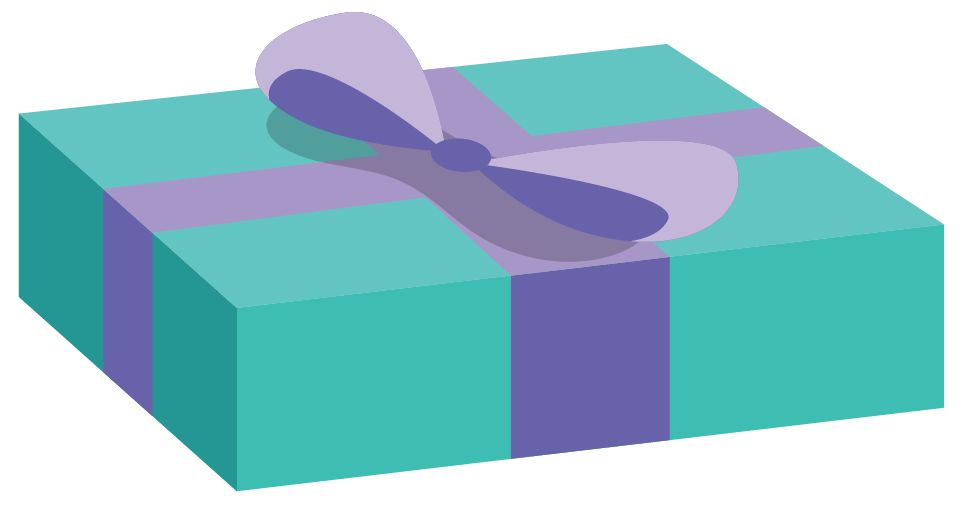Once you know the basic concepts and the various surface area formulae, you can calcuate the area of 3D shapes without a surface area calculator.

Check out the interactive simulations to know more about the lesson and try your hand at solving a few interesting practice questions at the end of the page.

## What is Surface Area?

Surface Area is the measure of the total area that the surface of a particular object occupies.

Real-life examples-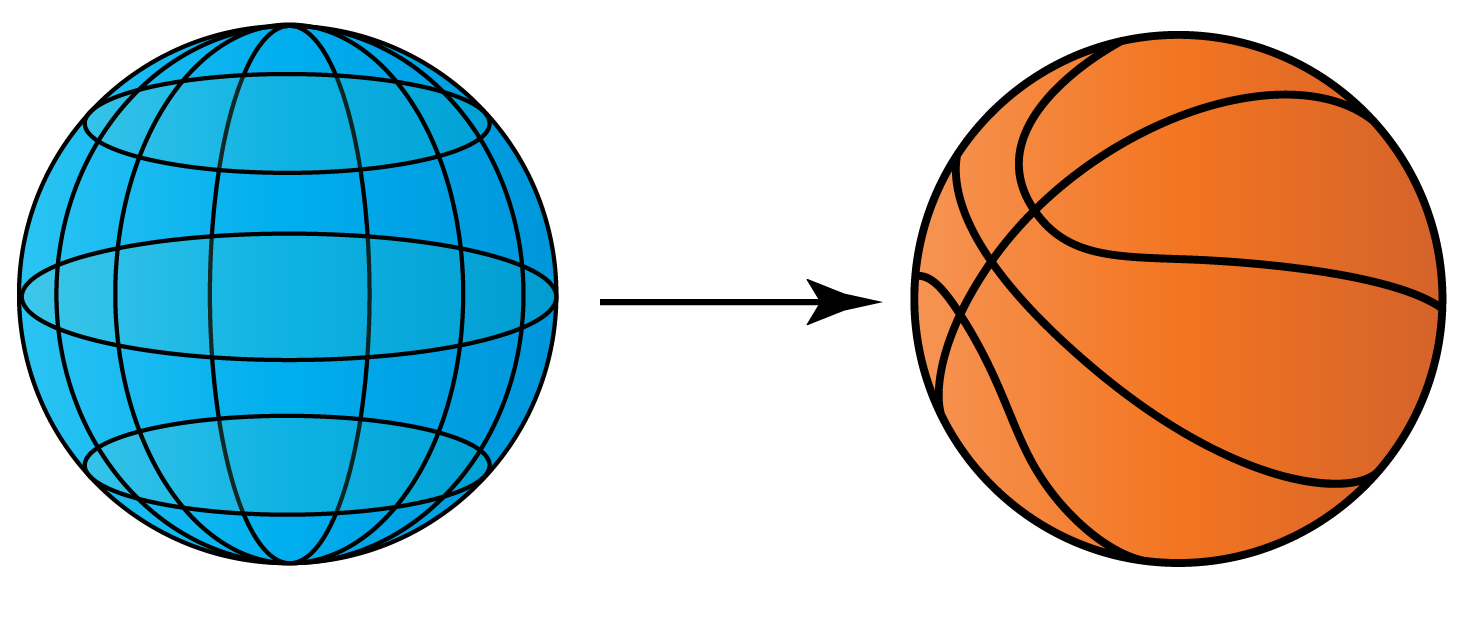Sphere - Ball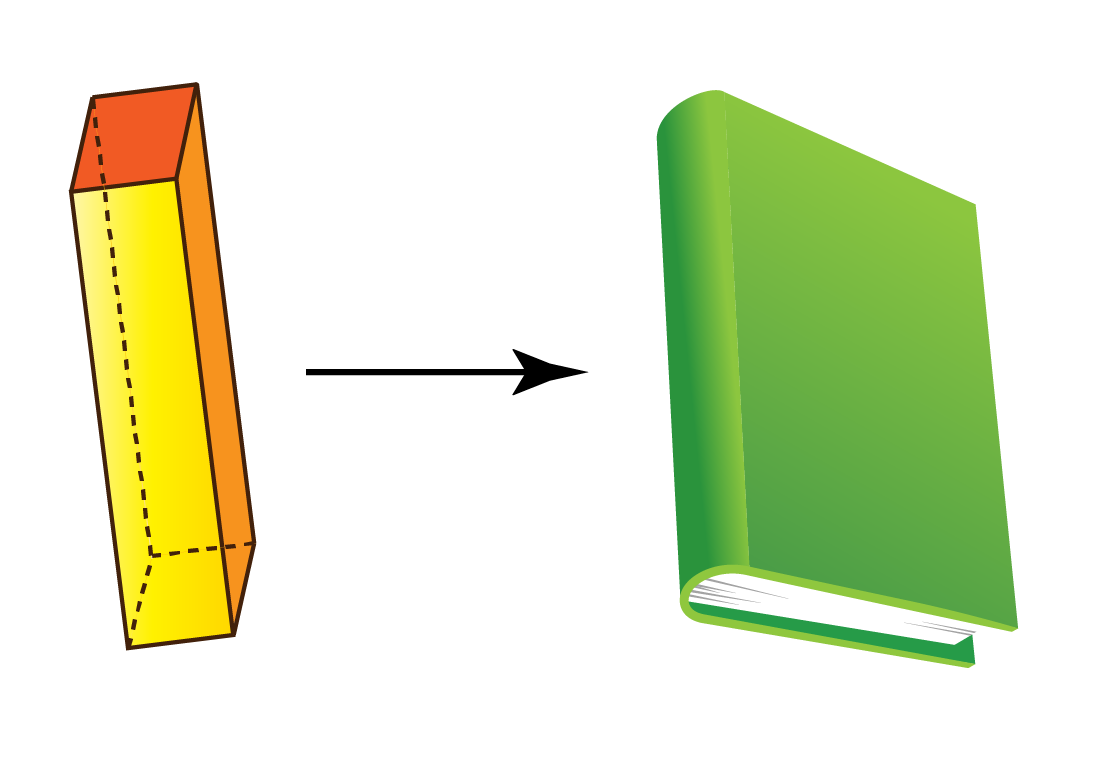Rectangular prism - Book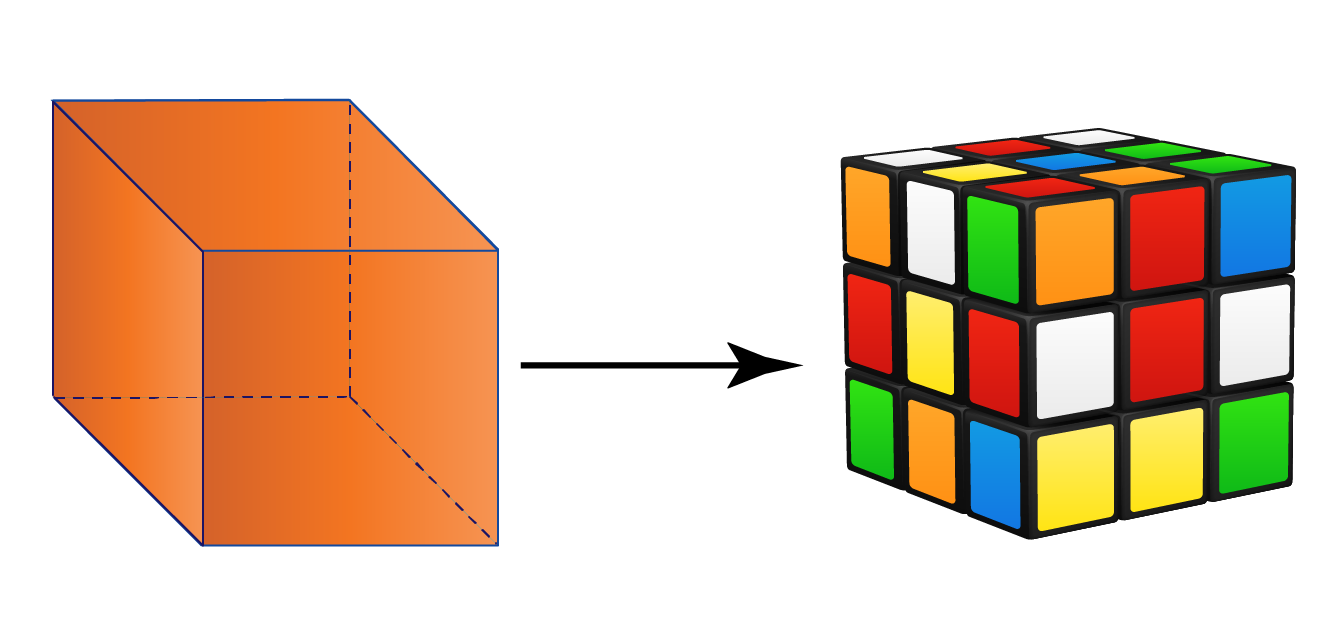Cube - Rubik's cube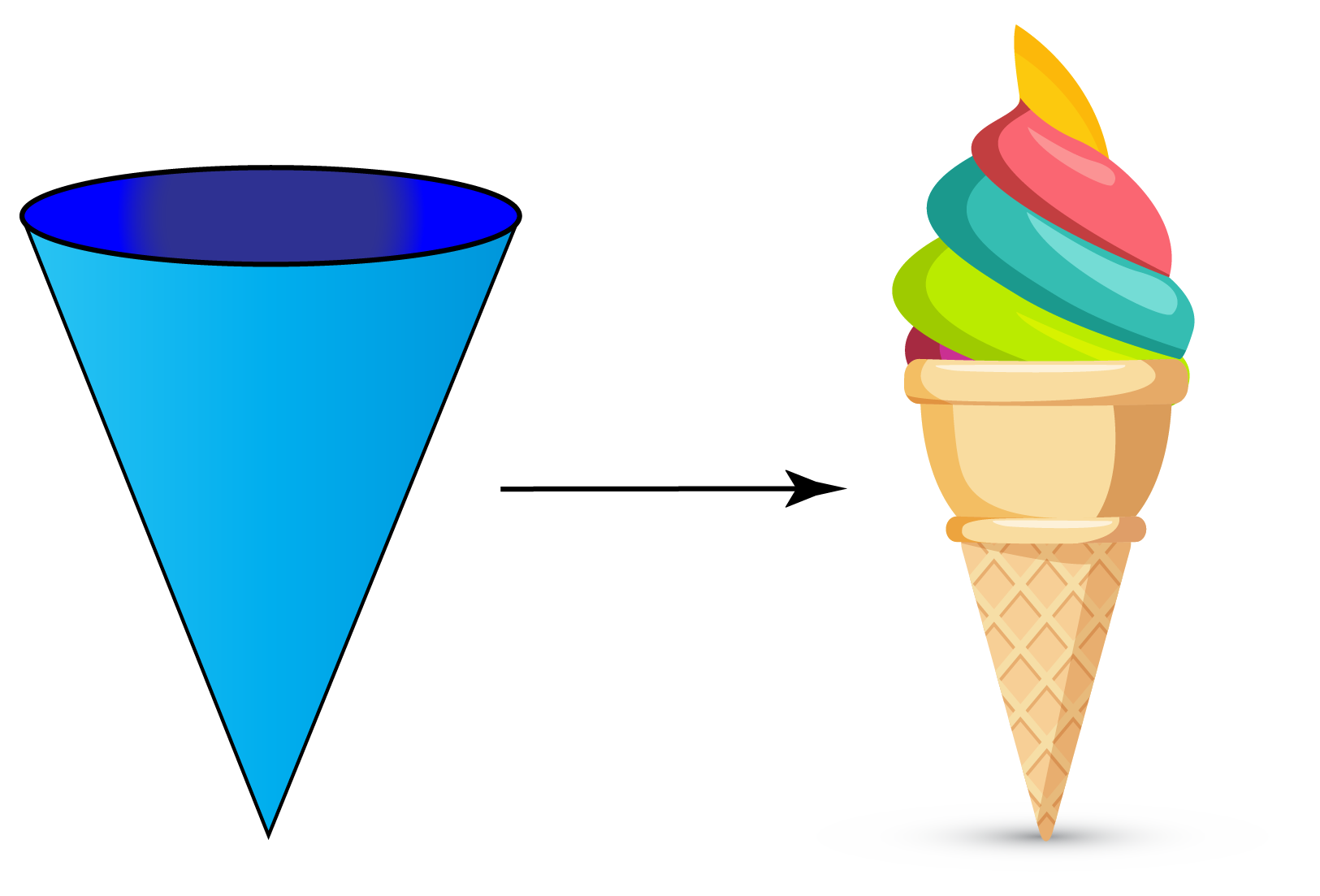Cone - Ice cream cone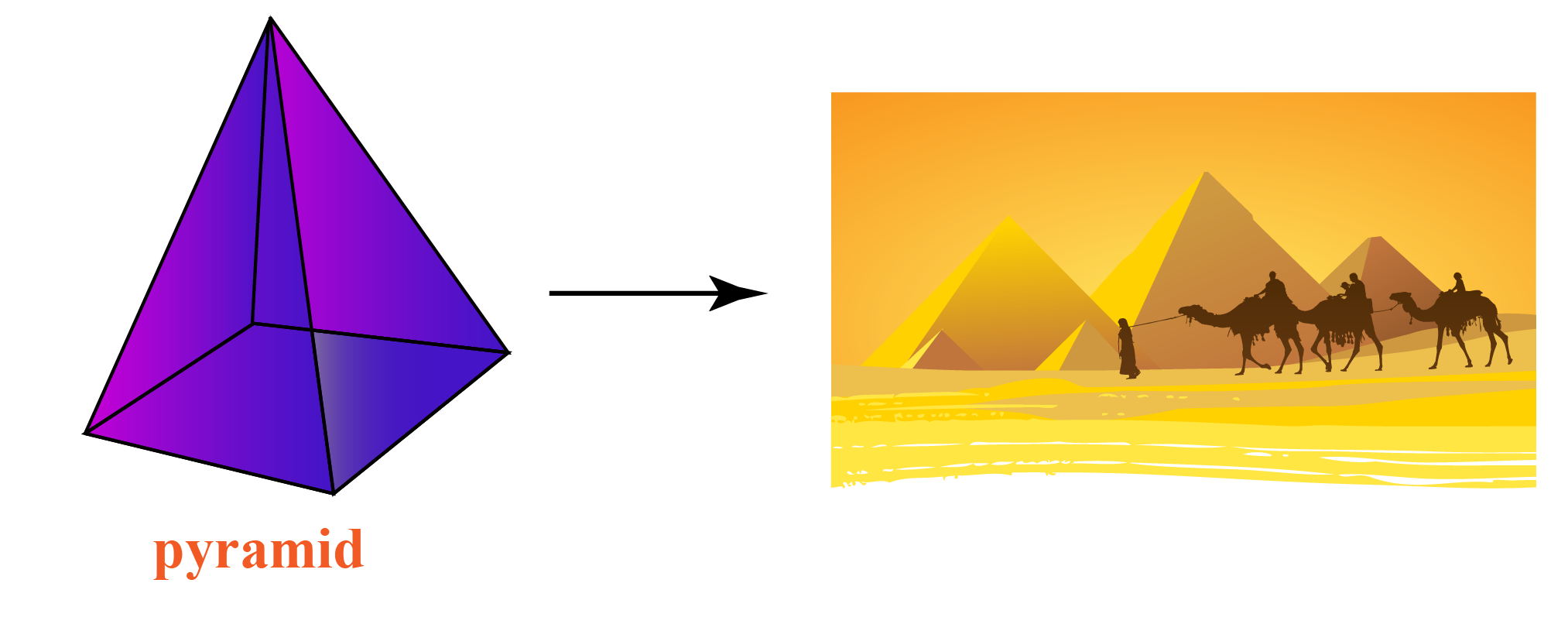Pyramid - Pyramids in Egypt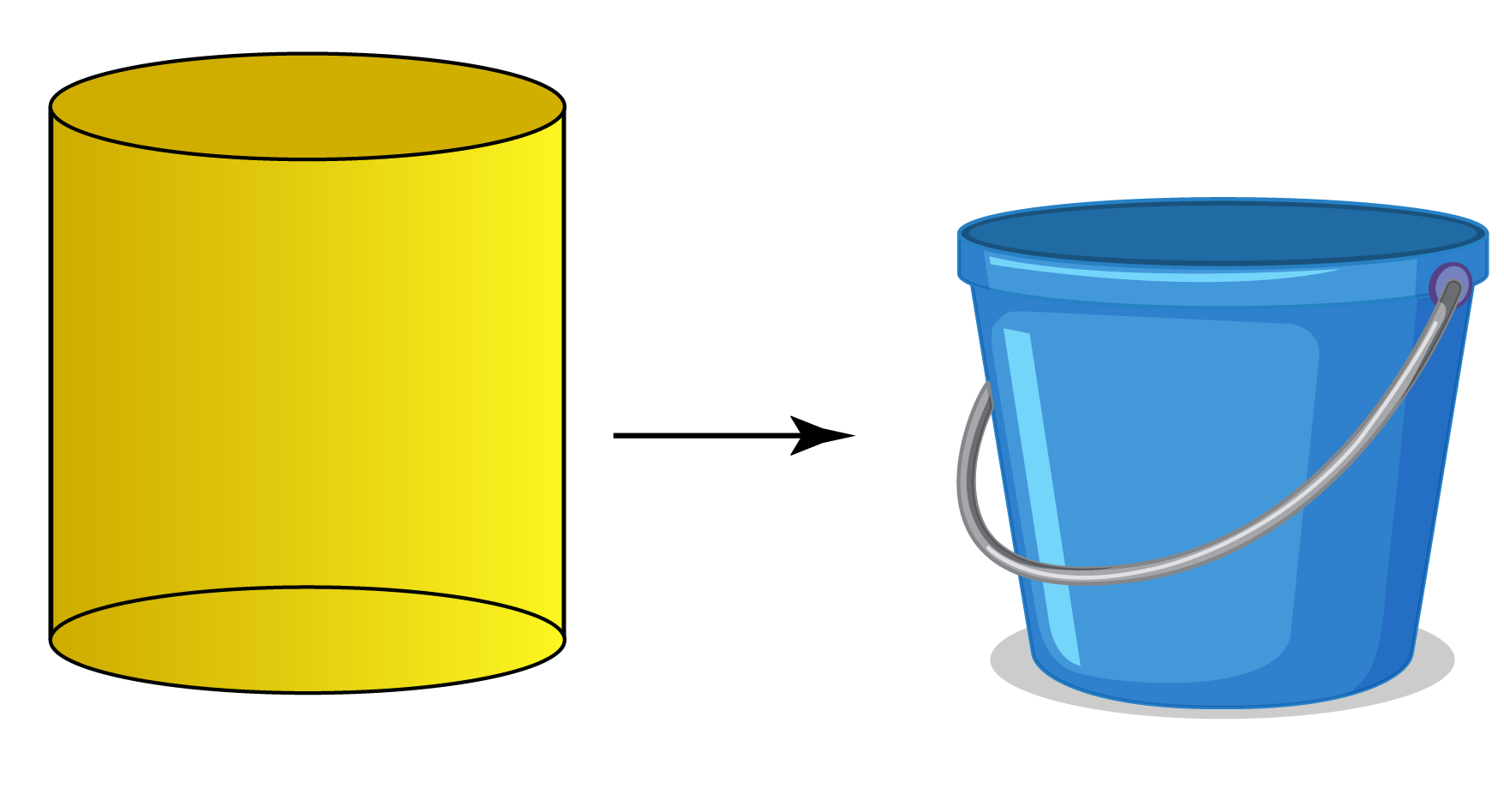Cylinder - BucketTips and Tricks

1. Do you know a cube is also known as hexahedron because of its $$6$$ identical square faces. So, whenever you are asked to find the surface area of a cube, just multiply the square of a side with $$6$$.

2. Three-dimensional objects have $$3$$ dimensions, namely length, breadth, and height.

3. $$3$$ D shapes have faces, edges, and vertices.

4. Surface area is classified into:

1. Curved surface area

2. Lateral surface area

3. Total surface area

## How to Find Surface Area

In simple words, the area of the whole of anything, whether it's an object or a surface, is the sum of the area of its constituent parts.

The surface area of a three-dimensional object is the total area of all of its surfaces.

The surface area is important to know in situations where we want to wrap something, paint something, or build things to get the best possible design.

## What is the General Formula of Surface Area of Sphere, Cylinder, Cone, Cube, Pyramid, Rectangular Prism, Triangular Prism.

### General Formula of Surface Area of Sphere-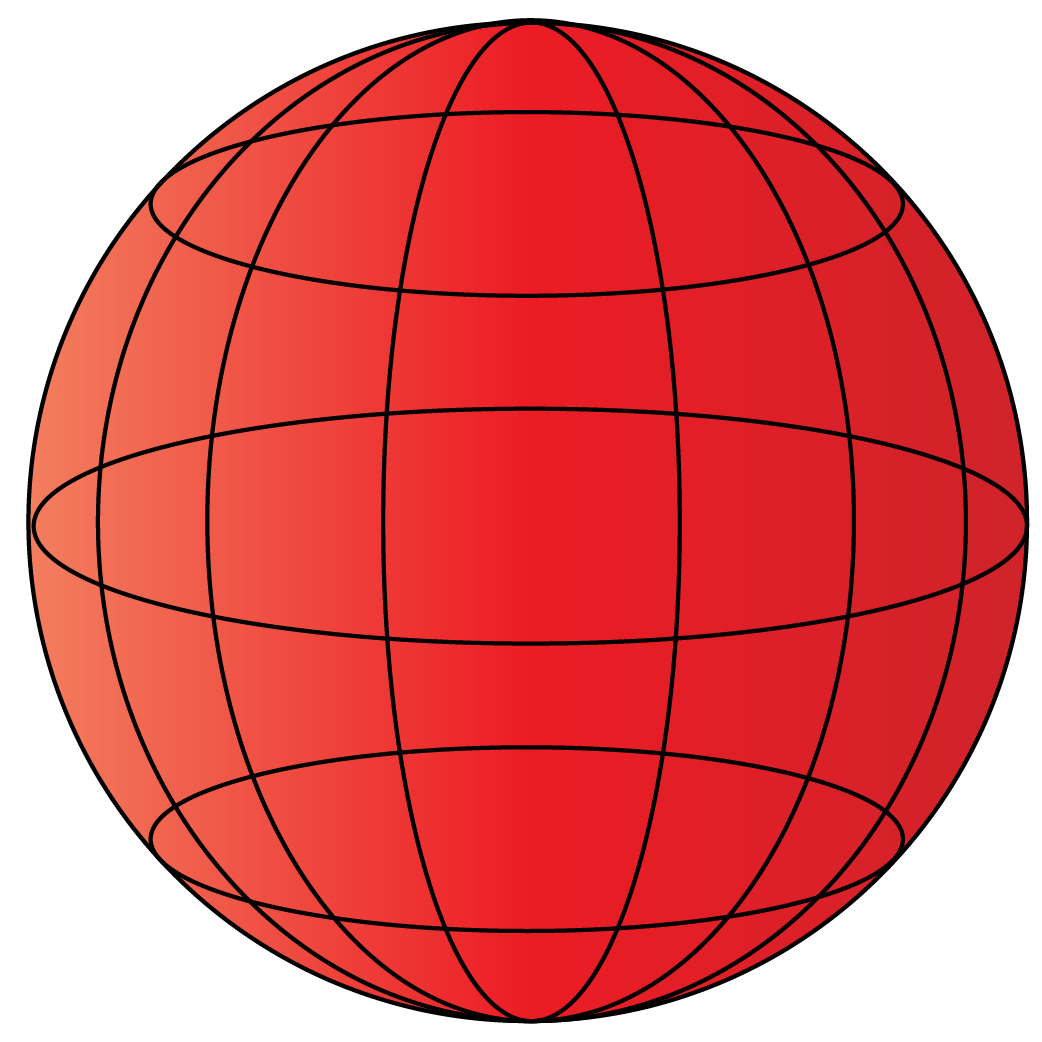The surface area of the sphere is $$4\pi r^{2}=\pi d^{2}$$

Where, $$r$$ = radius of  the sphere.

$$d$$ = diameter

### Formula of Surface Area of Cylinder-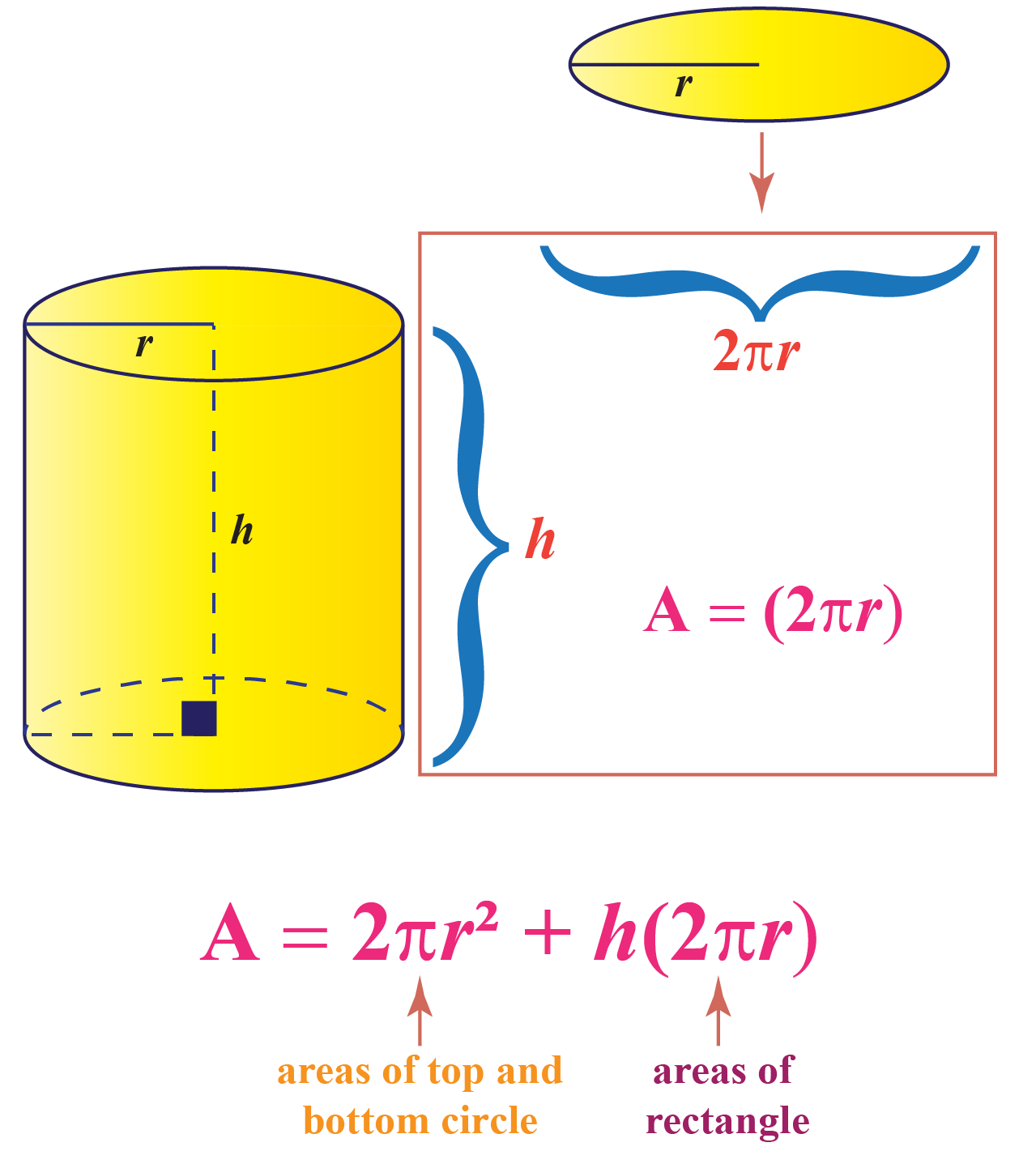The surface area of cylinder is $$2\pi r^{2}+2\pi rh = 2\pi r\left ( r+h \right )$$

Where, $$r$$ = radius of the cylinder.

$$h$$ = height of the cylinder

### Formula of Lateral Surface Area of Cone-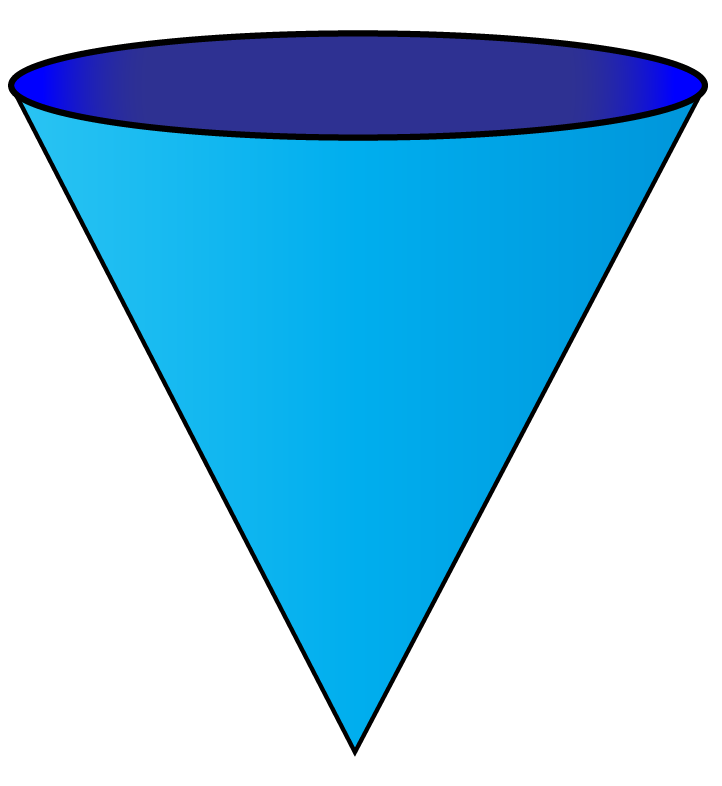$$\pi r\left ( \sqrt{r^{2}+h^{2}} \right )=\pi rs$$

Where,$$r$$ = radius of  the circular base.

$$h$$ = height of the cone

and $$s$$ = slant height of cone

### Formula of Total Surface Area of Cone-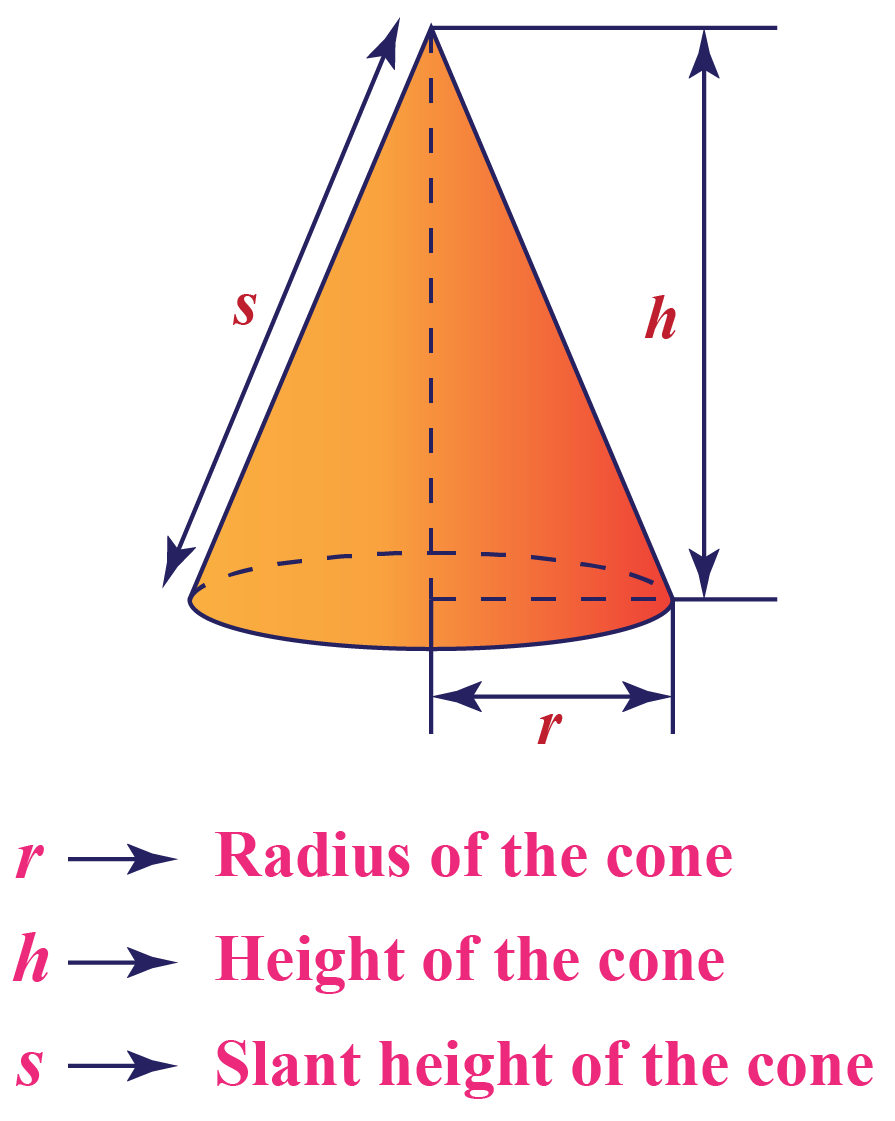$$\pi r\left ( r+\sqrt{r^{2}+h^{2}} \right )=\pi r\left ( r+s \right )$$

Where, $$r$$ = radius of  the circular base

$$h$$ = height of the cone

and $$s$$ = slant height of cone

### Formula of Surface Area of Pyramid-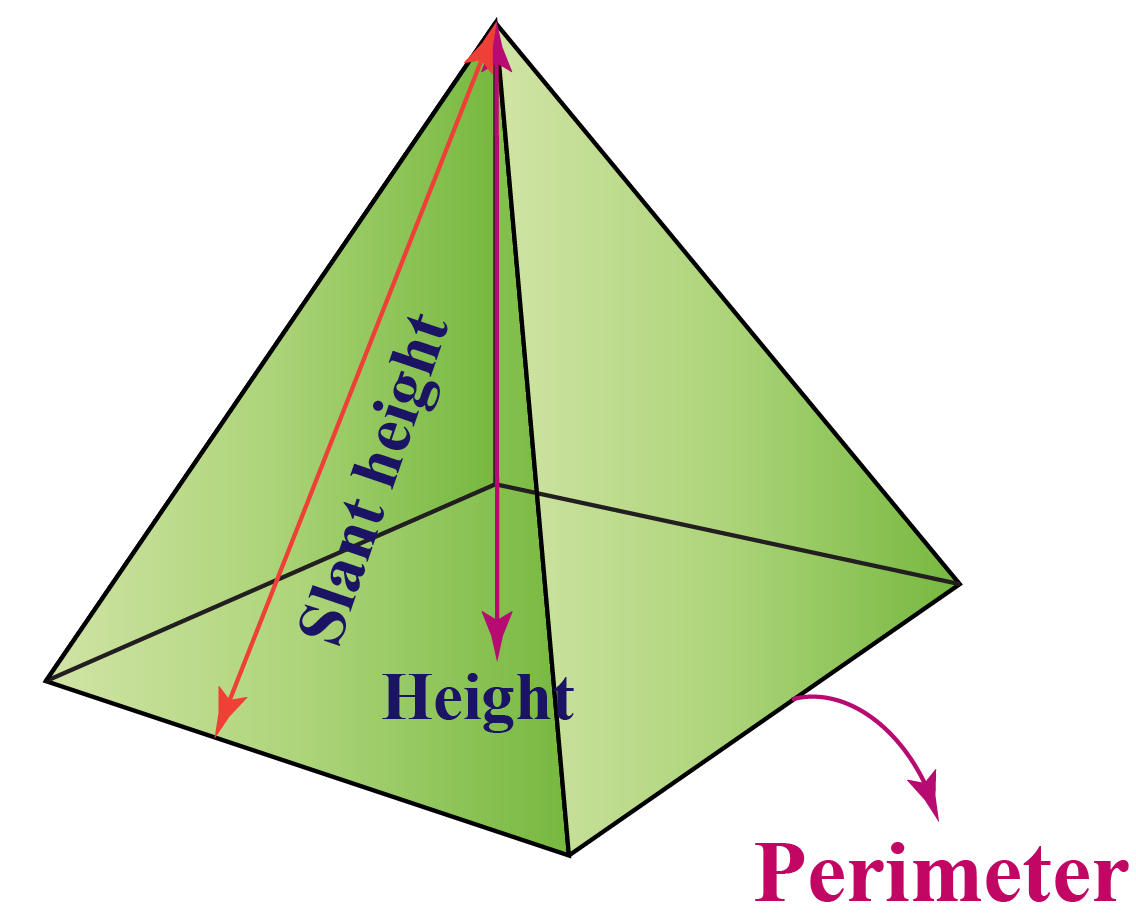$$B+\left ( \frac{PL}{2} \right )$$

Where, $$B$$ = area of  base.

$$P$$ = perimeter of base

and $$L$$ = slant height

##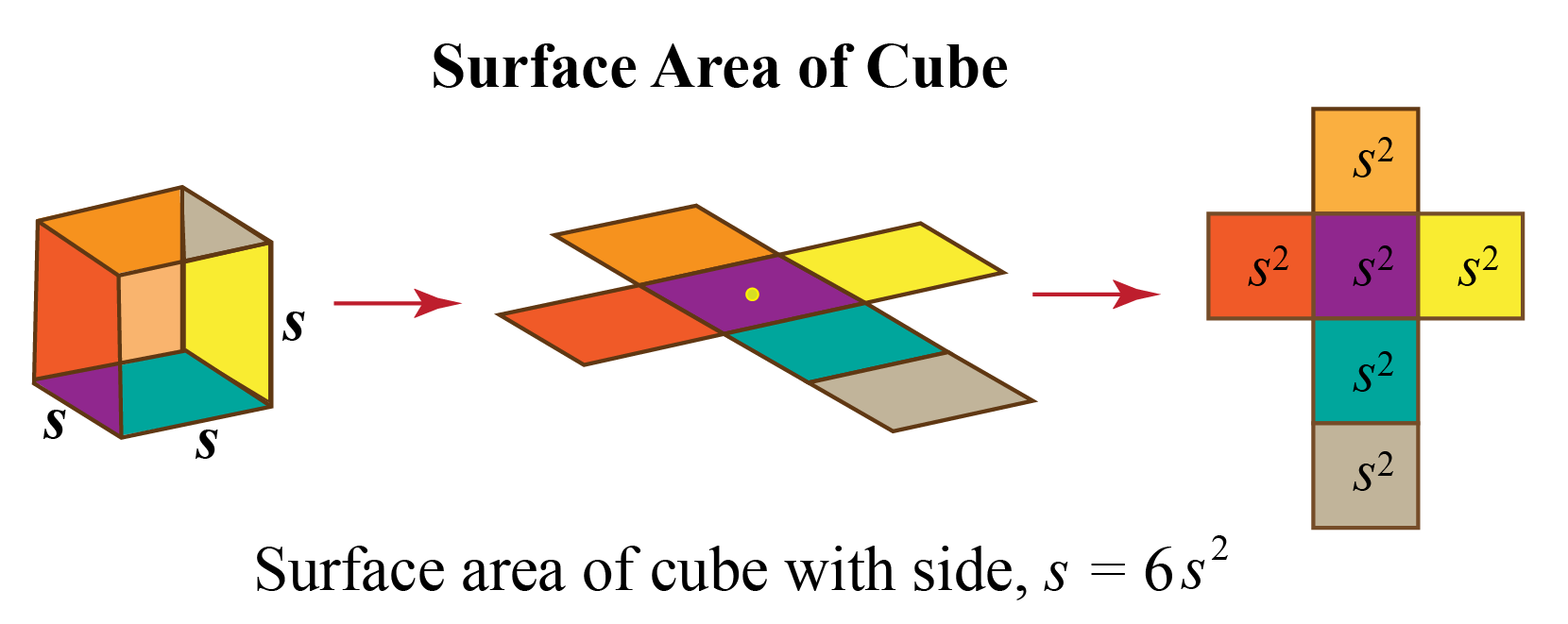$$6s^{2}$$

Where, $$s$$ = side length

### Formula of Surface Area of Triangular Prism-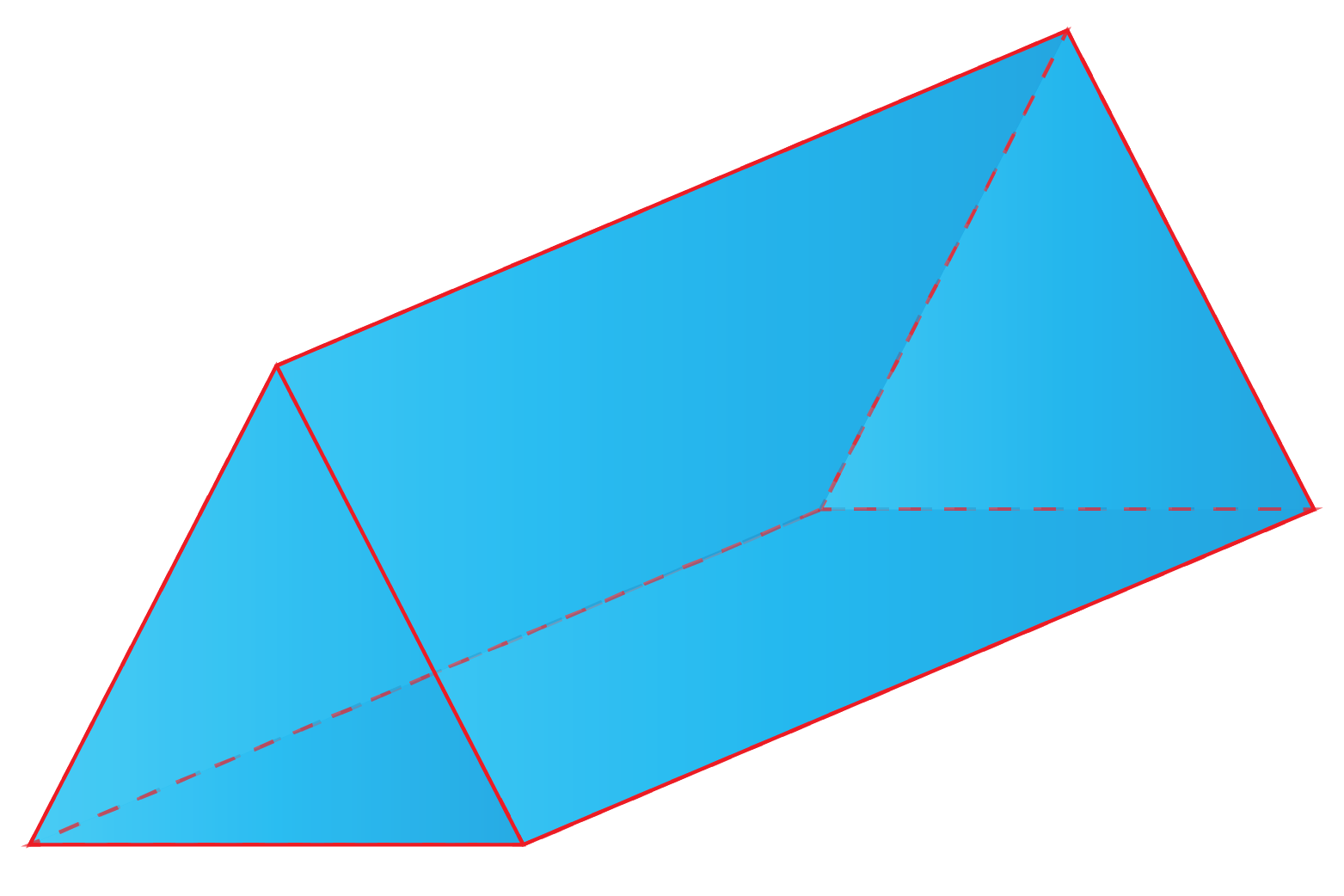$$bh+l\left ( a+b+c \right )$$

Where, $$b$$ = base length of triangle

$$h$$ = height of triangle

$$l$$ = distance between triangular bases

$$a,b,c$$ = sides of triangle

##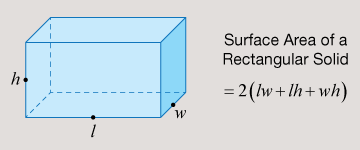$$A=2wl+2lh+2hw$$ or $$A=2\left ( wl+lh+hw \right )$$

Where, $$w$$ = width of the prism

$$h$$ = height of the prism

$$l$$ = length of the prismChallenging Questions
1. For her birthday, Emily wanted to make birthday caps in the form of right circular cones for all her friends.

She made 10 cones of base radius $$7$$ inches and height $$24$$ inches each.

Find the area of the sheet required to make $$10$$ such caps for her friends.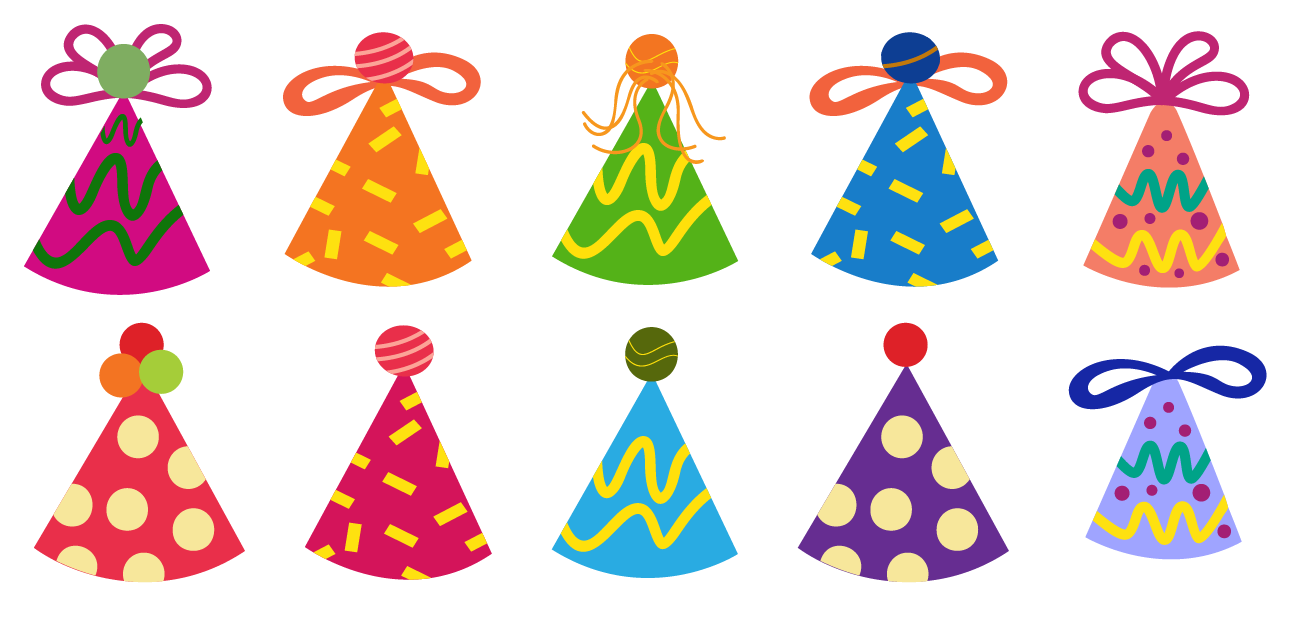## The Formula of Different Surface Areas with Sample Problems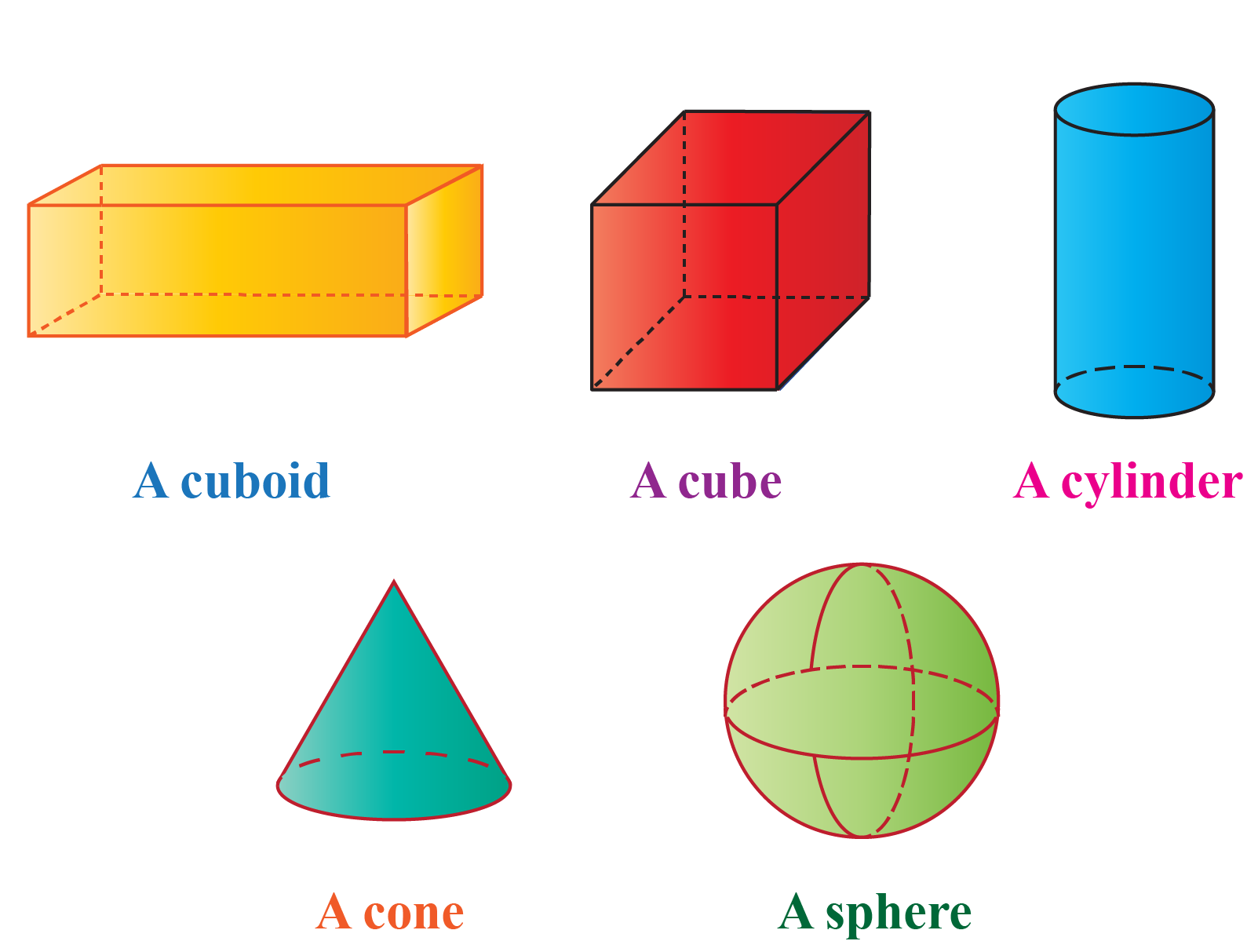### The surface area of a cone with a sample problem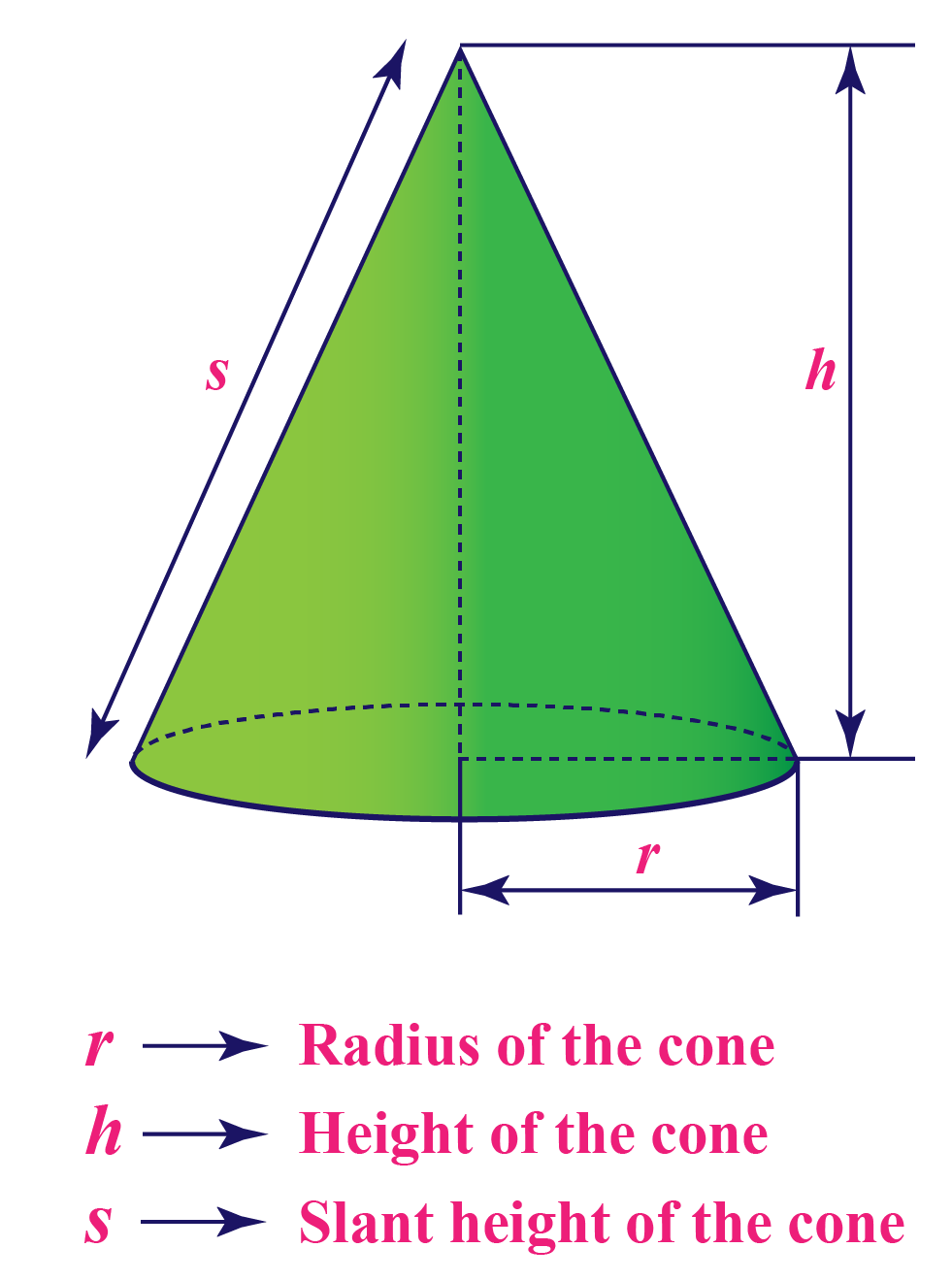Surface area of cone is  $$\pi r\left ( r+\sqrt{r^{2}+h^{2}} \right )=\pi r\left ( r+s \right )$$

Where, $$r$$ = radius of  the circular base.

$$h$$ = height of the cone

and $$s$$ = slant height of cone

 Example 1

Dylan observed the cone of the ice cream he was eating. To understand the concept and shape of a cone,

his father suggested that he would help him find the total surface area of the cone. Given that the radius = $$4$$ inches and height = $$7$$ inches.

what would be the surface area of the ice cream cone?

Solution

Given:

Radius = $$4$$ inches and height =$$7$$ inches

Surface area of cone

\begin{align} &= \pi rh+\pi r^2\\&=3.14\times 4\times 7+3.14\times 4^2\\&= 87.92 + 50.24\\&= 138.16 \text{ inches}^2\end{align}

 $$\therefore$$The surface area will be  $$138.16$$ $$inches^{2}$$

### The surface area of a cylinder with a sample problem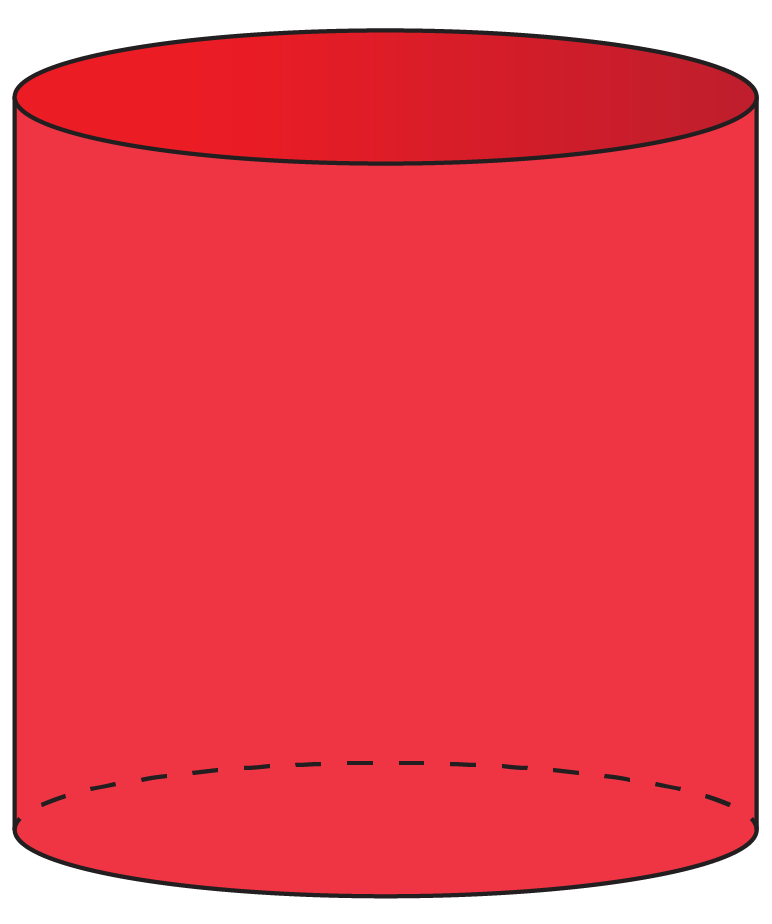The surface area of the cylinder is $$2\pi r^{2}+2\pi rh = 2\pi r\left ( r+h \right )$$

Where, $$r$$ = radius of  the cylinder.

$$h$$ = height of the cylinder

 Example 1

While painting a cylindrical tank of radius $$3.5$$ yd and height $$6$$ yd, Ryan thought of painting the outer surface.

If the cost of the painting is \$ $$5$$ per $$yd^{2}$$, what will be the total cost of painting?

Solution

Total Surface Area = Curved Surface Area + area of top and bottom faces
\begin{align} &=2\pi rh + 2\pi r^2 = 2πr (r + h)\\ &=2 × 22/7 × 3.5 × 3.5 + 6\\ &=22 × 9.5\\&= 209 \text{yd}^2\end{align}
Cost of painting at \begin{align} 5\text{ per yd}^2=209\times 5=1045\end{align}

 $$\therefore$$Cost of painting =$$1045$$

### The surface area of a cube with a sample problem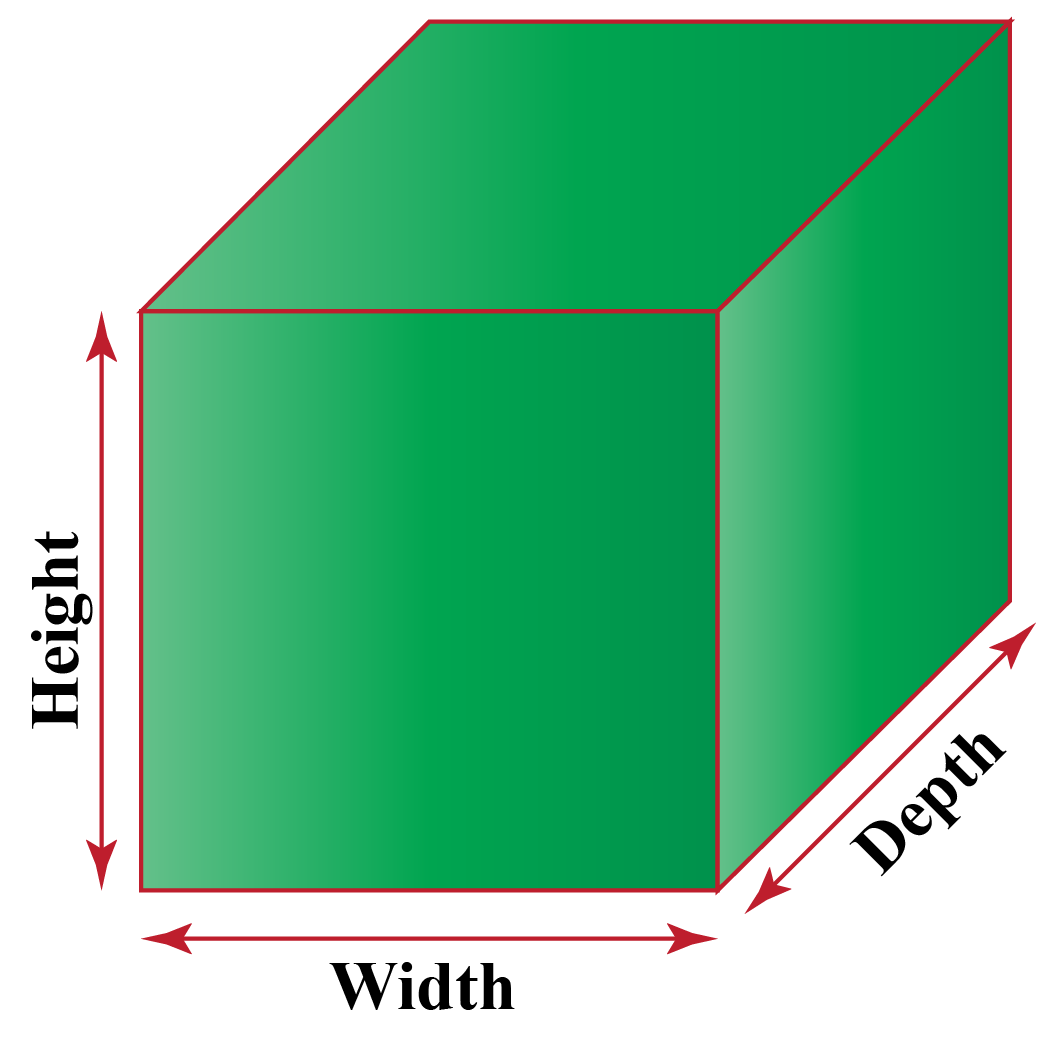The surface area of the cube is $$6s^{2}$$

Where, $$s$$ = side length

 Example 1

While playing with a Rubik's cube of side length $$3 inches$$, Antonia closely observed the shape that the shape resembled a cube that she learn about while learning shapes in school,

she thought of calculating its surface area to get a practical idea. What would be the surface area of the cube?

Solution

Given,

Side length \begin{align}&=3\text{inches}\\&=6s^{2}\\&=6\times 3^2\\&=6\times 9\\&=54\text{inches}^2\end{align}

 $$\therefore$$The surface area of cube will be  $$54 inches^{2}$$

### The surface area of cuboid with a sample problem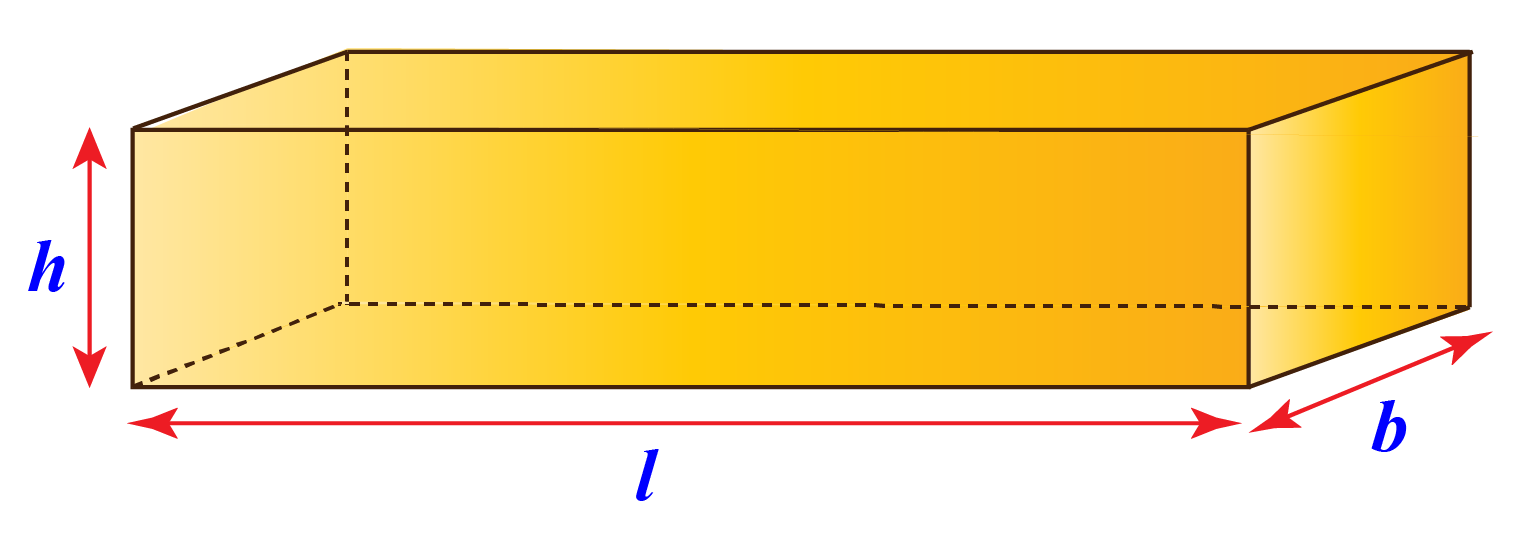The surface area of a cuboid $$2×(lb+bh+lh)$$
 Example 2

Ricky ordered a toy for his son from an online store. When the package came, he noticed that the box was a bit larger than the regular size for such a toy package.

If the length, width, and height of the box are $$7$$ inches, $$8$$ inches, and $$6$$ inches respectively, find the surface area of the cuboid-shaped box.

Solution

Given that,

Length of the cuboid = $$7$$ inches

Breadth of the cuboid = $$8$$ inches

Height of the cuboid = $$6$$ inches

Surface area of the cuboid is

\begin{align}&= 2×(lb+bh+lh)\\&=2\left ( 7\times 8+8\times 6+6\times 7 \right)\\&=2(56+48+42)\\ &= 2(146)\\&=292\text{ inches}^2\end{align}

 $$\therefore$$The surface area will be $$292 inches^{2}$$

### The surface area of a triangular prism with a sample problem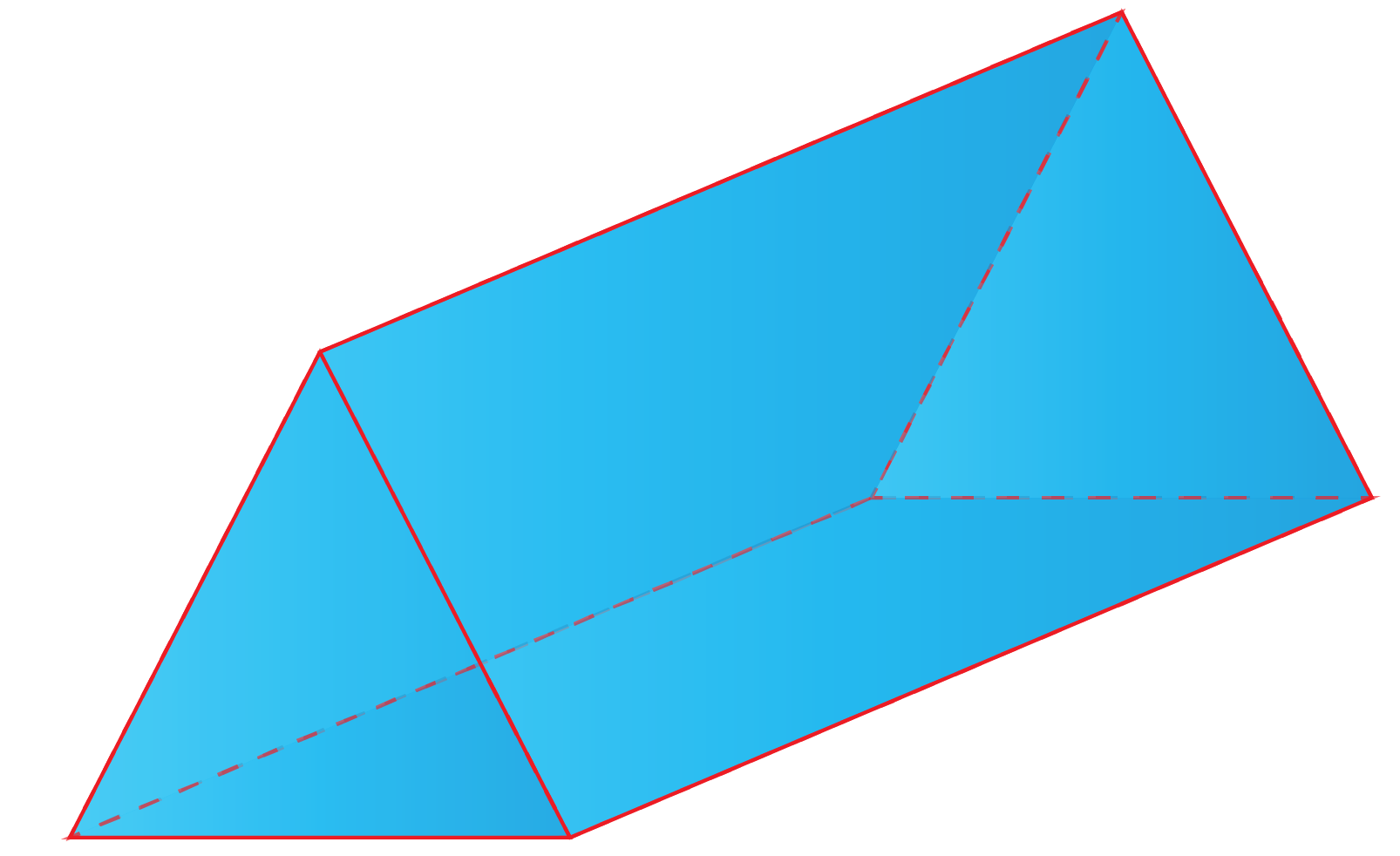$$bh+l\left ( a+b+c \right )$$

Where, $$b$$ = base length of triangle

$$h$$ = height of triangle

$$l$$ = distance between triangular bases

$$a,b,c$$ = sides of triangle

 Example 1

Ricky was curious to know the surface area of his kaleidoscope, so he asked his teacher who explained it to him.

The teacher gave an example of the surface area of a triangular prism with base $$4$$ ft, height $$3$$ ft, the distance between triangular bases $$10$$ ft, and sides a $$8$$ ft, b $$4$$ ft, c $$6$$ ft.

What would be the surface area of the kaleidoscope?

Solution

Given,

$$b$$ = $$4$$ ft

$$h$$ = $$3$$  ft

$$l$$ = $$10$$ ft

$$a$$ = $$8$$  ft

$$b$$ = $$4$$  ft

$$c$$ = $$6$$  ft

Surface area of the triangular prism:

\begin{align}&=bh+l\left ( a+b+c \right )\\&= 4\times 3+10\left ( 8+4+6 \right) \\&= 12+40+60+80\\&= 192\text{ ft}^2\end{align}

 $$\therefore$$The surface area of a triangular prism will be $$192 ft^{2}$$.

## Solved Examples of Surface Area

 Example 1

Stuart has a Rubik's cube with each side length = 5 inches. Can you help Stuart understand how to find the surface area of a cube by solving the question?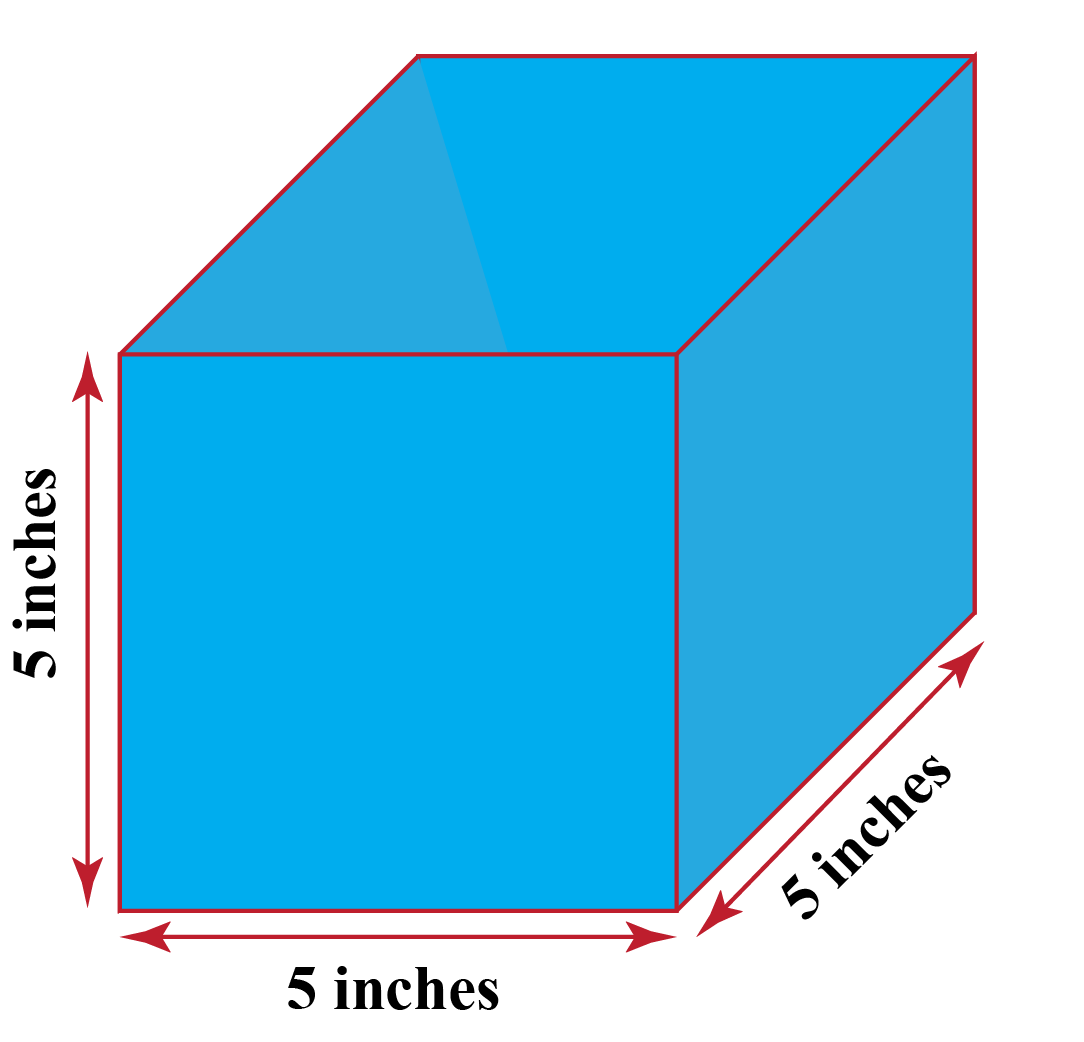Solution

Given,

Length of side of the cube = a = $$5$$ inch

Surface area of cube

\begin{align}&= 6a^2\\ &= 6\times 5^2\text{inch}^2\\ &= 6\times 25 \text{inch}^2\\&= 150 \text{inch}^2\end{align}

 $$\therefore$$The surface area of the cube will be $$150$$ $$inch^{2}$$
 Example 2

Wilson gave a sphere-shaped balloon to his son. The balloon had some chocolates inside it. To test his son’s knowledge, Wilson asked his son to calculate the total surface area of the balloon (given that the radius is $$12 inches$$).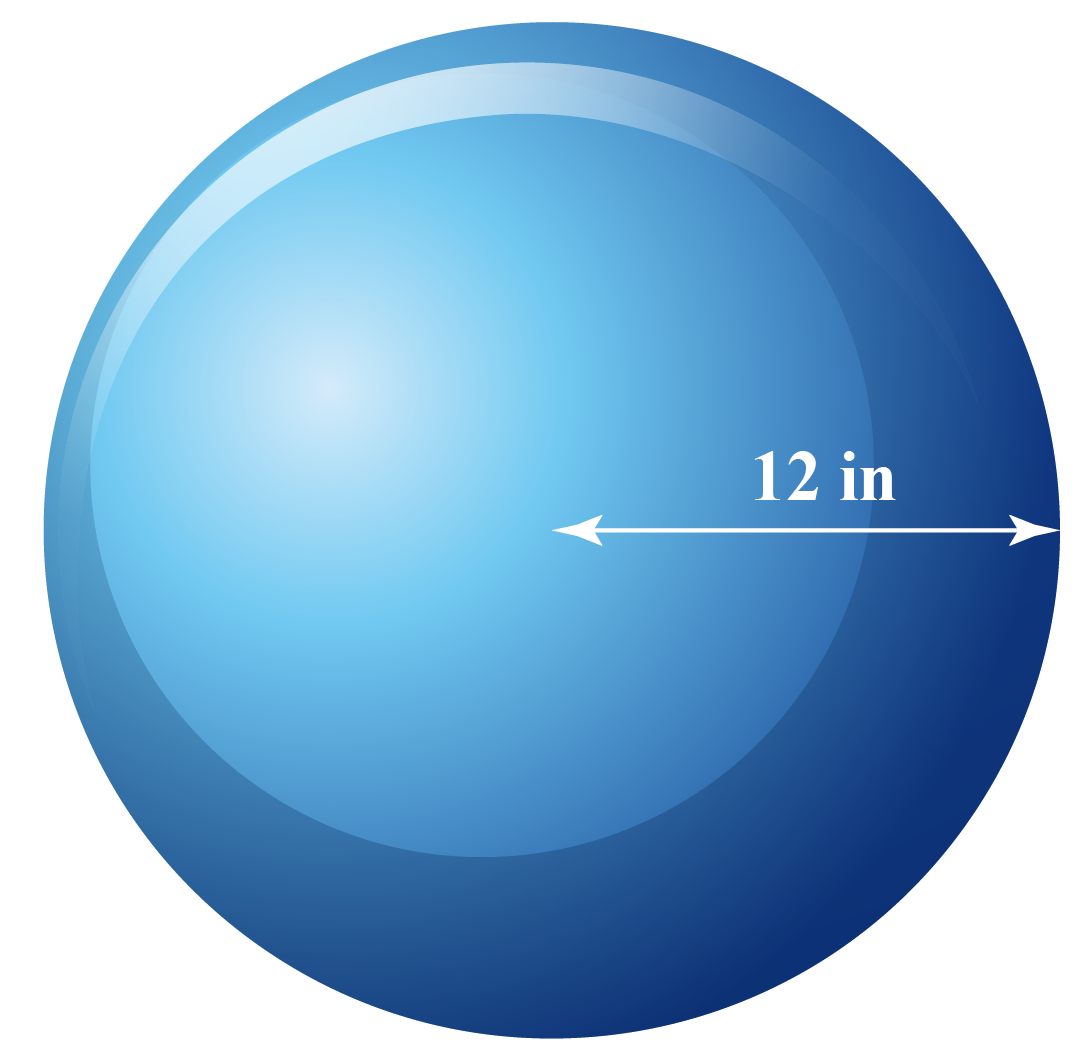Solution

Given,

Radius of the sphere = $$12 inch$$

Surface area of the sphere

\begin{align}&=4\pi r^2\\&= 4\pi \left (12^2 \right)\\&= 4\pi \left ( 144 \right)\\&= 576\pi\text{ or}576\times 3.14\text{ inch}^2\end{align}

 $$\therefore$$The surface area will be  $$576\pi$$ or $$576\times 3.14 inches^{2}$$.
 Example 3

Help Kaley understand how to find the surface area of a cylinder with diameter $$12$$ inch and height $$8$$ inch.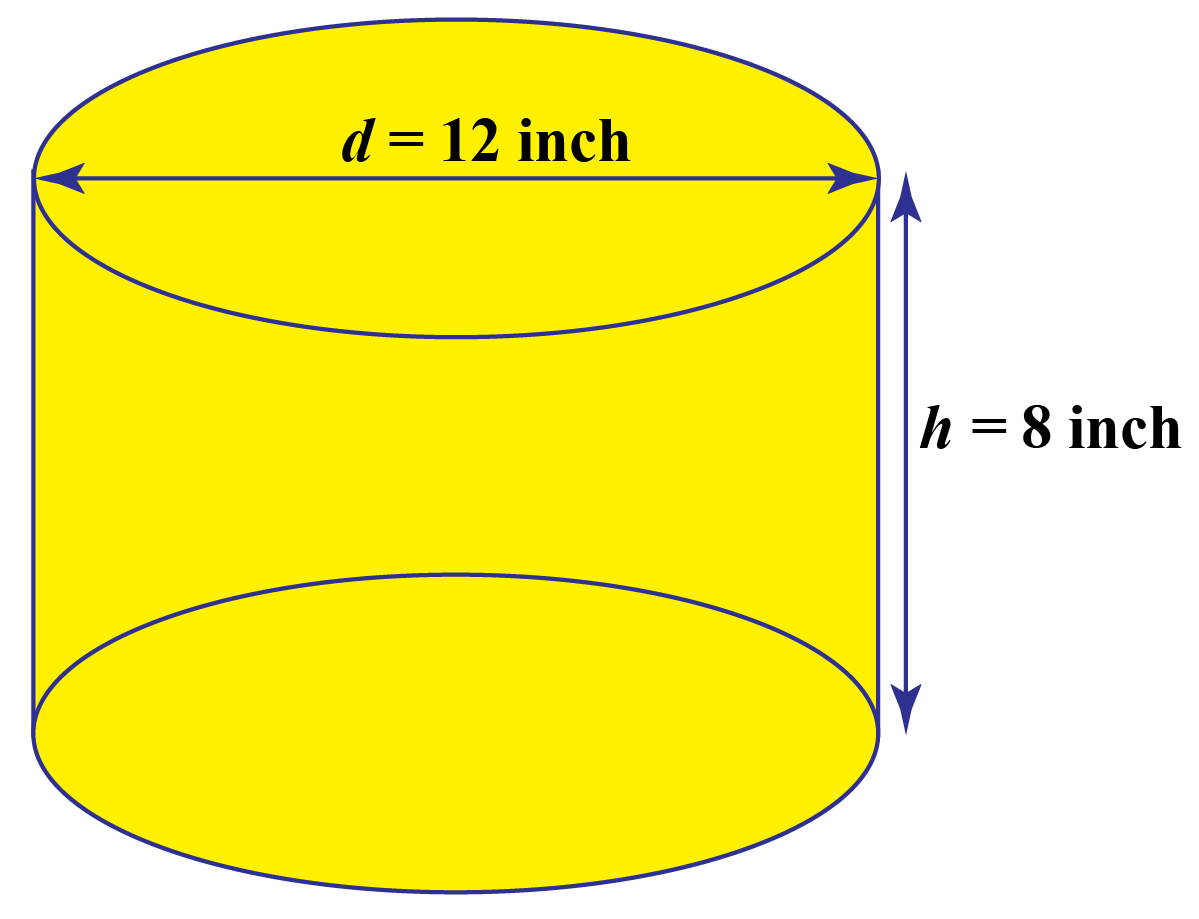Solution

Given,

Diameter = $$12$$ inch so radius will be,

$$r=\frac{d}{2}$$ i.e. $$r=\frac{12}{2} = 6 inch$$

Surface area of cylinder

\begin{align}&= 2\pi r\left ( r+h \right)\\&= 2\times \dfrac{22}{7}\times 6\left ( 6+8 \right)\\&= 2\times \dfrac{22}{7}\times 6\left ( 14 \right)\\&= 2\times \dfrac{22}{7}\times 84\\&= 2\times 22\times 12\\&= 528 \text{inch}^2\end{align}

 $$\therefore$$The surface area of the cylinder will be   $$528$$ $$inch^{2}$$.
 Example 4

Find the surface area of a pyramid with a square base of edge $$16$$ inch, slant height $$17$$ inch, and altitude $$15$$ inch.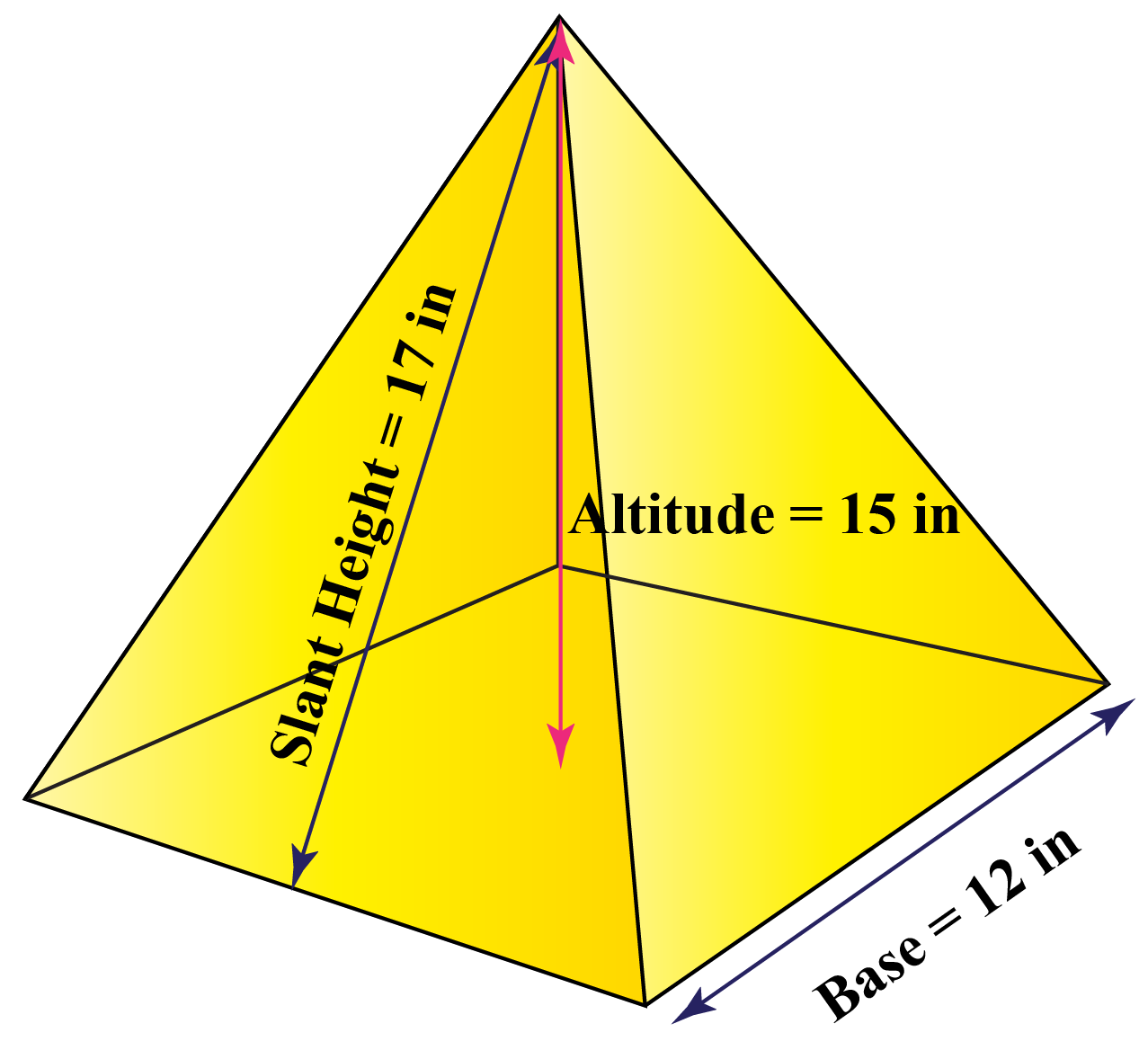Solution

Given,

base length = $$12$$ inch.

So perimeter of base will be

\begin{align}P& = 4s\\P &= 4\times 12=48\text {inches}\end{align}

Area of base is $$s^{2}$$

\begin{align}B& = 12^2\\&= 144\text{ inches}^2\end{align}

Total surface area

\begin{align}&= B+\frac{PL}{2}\\&= 144+\frac{48\times 17}{2}\\&= 144+\frac{816}{2}\\&= 144+408\\ &= 552 \text{ inches}^2\end{align}

 $$\therefore$$The surface area will be $$552$$  $$inches^{2}$$
 Example 5

Can you help Sophia find the surface area of a rectangular prism whose width is $$30$$ inch, the length is $$15$$ inch, and height is $$20$$ inch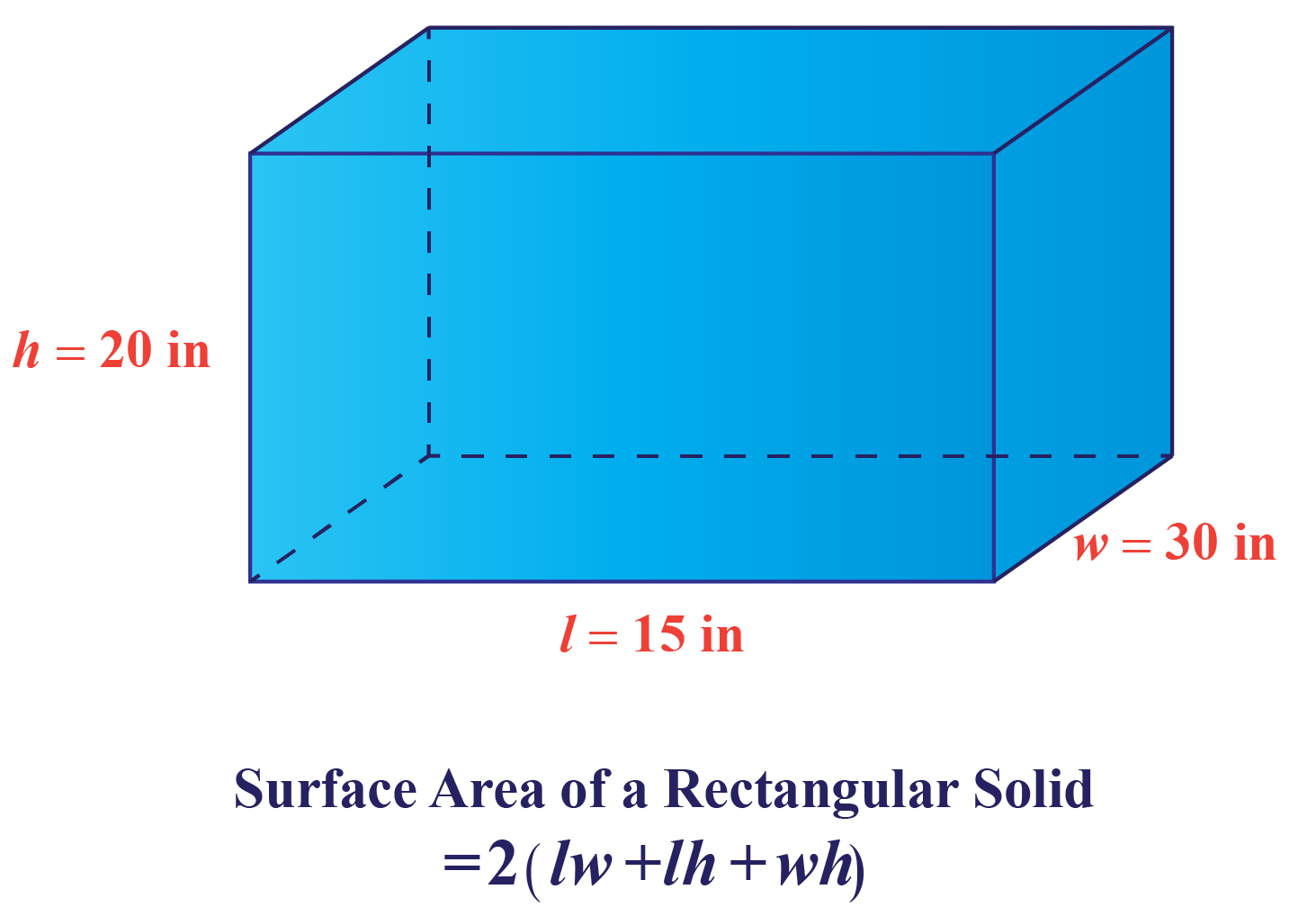Solution

Given,

$$W$$ = $$30 inch$$

$$L$$ = $$15 inch$$

$$H$$ = $$20 inch$$

\begin{align}A &= 2\left ( WL+LH+HW \right )\\A &= 2\left ( 450+300+600 \right )\\ &= 2\left ( 1350 \right )\\&= 2700\text{ inch}^2\end{align}

 $$\therefore$$The surface area of the rectangular prism will be  $$2700$$ $$inch^{2}$$

Surface Area Calculator

Talking about the surface area calculator, it helps you find the surface area of several shapes.

It simply works like any other calculator in which you put the input in the form of dimensions and

it will put it in the place of the formula and you will get the answer

## Let's Summarize

We hope you enjoyed learning about how to find the surface area with the simulations and practice questions. Now you will be able to easily solve problems on the surface area, surface area formula, surface area calculator, how to find the surface area of a rectangular prism, how to find the surface area of the cylinder

At Cuemath, our team of math experts is dedicated to making learning fun for our favorite readers, the students!

Through an interactive and engaging learning-teaching-learning approach, the teachers explore all angles of a topic.

Be it worksheets, online classes, doubt sessions, or any other form of relation, it’s the logical thinking and smart learning approach that we, at Cuemath, believe in.

## 1. Is surface area the same as area?

The main difference is that surface area is the area of all the constituent parts of  3D shapes such as a sphere, cylinder, etc. whereas area is the measurement of the size of a plane surface, i.e. a 2D shape such as triangle, square, etc.

##Let us take an example of a cuboid-shaped solid of length $$8$$ inches, breadth(width) $$6$$ inches, and height $$5$$ inches.

What will be the surface area of the cuboid?

Given, a = $$8$$ inches, b = $$6$$ inches, h = $$5$$ inches
The total surface area \begin{align}&= 2(ab + ah + bh)\\ & = 2 × [(8 × 6) + (5 × 6) + (8 × 5)]\\ &= 236\text { sq.inches}\end{align}

## 3. What is the surface area of a circle?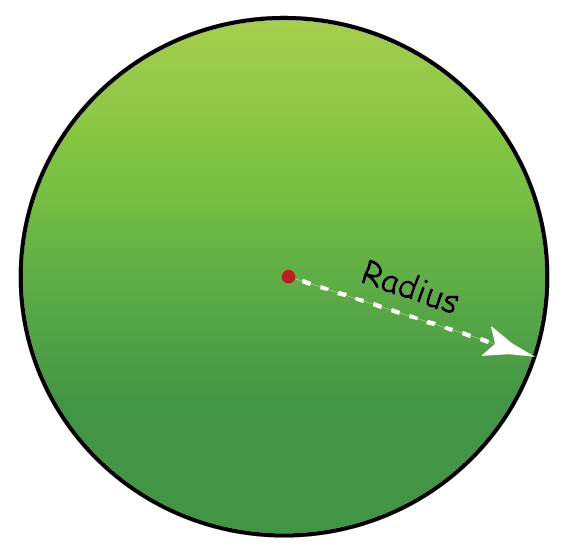The surface area of a circle is $$\pi r^{2}$$

More Important Topics
Numbers
Algebra
Geometry
Measurement
Money
Data
Trigonometry
Calculus